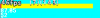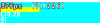# 3Dvertex_freeze() makes things worse

#### GrizzliusMaximus

##### Member
So I'm making a game that makes use of chunks like Minecraft. Each chunk has a vertex buffer and I thought of freezing the vertex buffer to reduce the lag but the opposite happened.

Without vertex_freeze()with vertex_freeze()Now it looks like vertex_freeze() is helping if you look at the bar and the fps_real (value under the bar) however, the actual fps is lower with vertex_freeze(). There is also more vertex batches.

I think the bottleneck is caused by my GPU and if I were to test this on a PC with better GPU, vertex_freeze() may actually double the framerate. I am running my game with Intel HD Graphics so clearly not the best graphics.

So... if my speculation is true, why is GPU having more trouble working on a frozen vertex buffer?

(Also, if you are questioning the high number of vertex batches, I'm over stressing the game for the sake of this thread. Normally, there will be about 25-30 vertex batches)

#### Nocturne

##### Friendly Tyrant
Forum Staff
Admin
Moderator
Could you explain how you are using the vertex_freeze() function? In fact, how the whole buffer creation and draw pipeline is structured?

•Sybok

#### GrizzliusMaximus

##### Member
At the start of the game, I setup the vertex format as so:
GML:
``````vertex_format_begin();
vertex_format_add_position_3d();
vertex_format_add_color();
vertex_format_add_textcoord();
v_format = vertex_format_end();``````
Then whenever I load a new chunk, I create the vertex buffer, construct it, save its id onto a list then freeze it (Only the world vertex buffer):
GML:
``````            var vb = vertex_create_buffer(); //world vertex buffer
var tvb = vertex_create_buffer(); //tree vertex buffer
var svb = vertex_create_buffer(); //tree shadow vertex buffer
vertex_begin(vb,_vf);
vertex_begin(tvb,_vf);
vertex_begin(svb,_vf);
random_set_seed(xxx+yyy);
for (var i = 1; i < 17; i++){
for (var j = 1; j < 17; j++){
var ht = cm2[|j+18*i]; // height of block

//height of adjacent blocks
var htd = cm2[|j+18*i+18];
var htu = cm2[|j+18*i-18];
var htr = cm2[|j+1+18*i];
var htl = cm2[|j-1+18*i];
var htdr = cm2[|j+1+18*i+18];
var htdl = cm2[|j-1+18*i+18];
var htul = cm2[|j-1+18*i-18];
var htur = cm2[|j+1+18*i-18];

//Position of block
var xx = j*32+xxx-32;
var yy = i*24+yyy-24;
var zz = -ht*8;
var sub = ceil(ht);
if (ht > 48){
sub = 5;
}
else if (ht > 32){
sub = 4;
}
else if (ht > 3){
sub = 3;
if (random(10) < 1){
//colors used to determine current corner in vertex shader
var c1 = \$ff00ff00;
var c2 = \$ffffff00;
var c3 = \$ff00ffff;
var c4 = \$ffffffff;
DrawQuadExt(tvb,spr_tree,3,xx-48,yy+19+((xx/32)%5)/10,zz-91,0,0,0,128,0,96,c1,c2,c3,c4); //tree sprite
DrawQuad(svb,spr_tree_shadow,3,xx-16,yy+3,0,0,0,0,64,24,0); // tree shadow
}
}
else if (ht > 1){
sub = 2;
}

//colors to add shadows based on adjacent blocks
var c1 = \$ffffffff;
var c2 = \$ffffffff;
var c3 = \$ffffffff;
var c4 = \$ffffffff;

var side = 0;
if (ht < htd){
c3 -= \$00111100;
c4 -= \$00111100;
side = 1;
}
if (ht < htu){
c1 -= \$00111100;
c2 -= \$00111100;
side = 1;
}
if (ht < htl){
c1 -= \$00111100;
c3 -= \$00111100;
side = 1;
}
if (ht < htr){
c2 -= \$00111100;
c4 -= \$00111100;
side = 1;
}
var sgn = 1;
if (side == 0){
if (ht < htdl && ht >= htdr && ht >= htur && ht >= htul){
c4 -= \$00111100;;
DrawQuadExt(vb,spr_floor,sub,xx+32,yy,zz,0,0,0,-32,24,0,c1,c2,c3,c4);
continue;
}
if (ht < htur && ht >= htdl && ht >= htdr &&  ht >= htul){
c1 -= \$00111100;
DrawQuadExt(vb,spr_floor,sub,xx+32,yy,zz,0,0,0,-32,24,0,c1,c2,c3,c4);
continue;
}

if (ht < htdr){
c4 -= \$00111100;
}
if (ht < htdl){
c3 -= \$00111100;
}
if (ht < htur){
c2 -= \$00111100;
}
if (ht < htul){
c1 -= \$00111100;
}

}

if (ht > htd){
DrawQuad(vb,spr_wall,sub,xx,yy+24,zz,0,0,0,32,0,(ht-htd)*8); //draw wall. Only one wall is visible since projection is orthographic
}

DrawQuadExt(vb,spr_floor,sub,xx,yy,zz,0,0,0,32*sgn,24,0,c1,c2,c3,c4); //draw floor

}
}
vertex_end(vb);
vertex_end(tvb);
vertex_end(svb);

cm[| 3] = vb;
cm[| 4] = tvb;
cm[| 5] = svb;
loaded_map[? key] = 1;

vertex_freeze(vb); //<------------ Vertex Freeze after vertex_end()``````
This code is only run once per loaded chunk. If it gets unloaded and reloaded, it will run this code again. During unloading, the vertex batch is deleted. Chunks are unloaded only when it is out of view region.

Then at every draw, the vertex buffer of every loaded chunk is rendered:
GML:
``````repeat(cm_s){ //cm_s = size of chunk_map
var cm = chunk_map[? key];
var vb = cm[| 3];
var tvb = cm[| 4];
if (vb != noone){
vertex_submit(vb,pr_trianglelist,tex); //drawing of the world vertex batch
tl[cnt--] = tvb; //save tree vertex batches to array to be rendered later
}
key = ds_map_find_next(chunk_map,key);
}``````

This is the code for DrawQuadExt():
GML:
``````var vb = argument0;
var spr = argument1;
var img = argument2;
var xx = argument3;
var yy = argument4;
var zz = argument5;
var x1 = argument6;
var y1 = argument7;
var z1 = argument8;
var x2 = argument9;
var y2 = argument10;
var z2 = argument11;
var c1 = argument12;
var c2 = argument13;
var c3 = argument14;
var c4 = argument15;

var tex = sprite_get_uvs(spr,img);
vertex_position_3d(vb,xx+x1,yy+y1,zz+z1);
vertex_argb(vb, int64(c1));
vertex_texcoord(vb,tex,tex);
vertex_position_3d(vb,xx+x2,yy+y1,zz+z1);
vertex_argb(vb, int64(c2));
vertex_texcoord(vb,tex,tex);
vertex_position_3d(vb,xx+x1,yy+y2,zz+z2);
vertex_argb(vb, int64(c3));
vertex_texcoord(vb,tex,tex);
vertex_position_3d(vb,xx+x1,yy+y2,zz+z2);
vertex_argb(vb, int64(c3));
vertex_texcoord(vb,tex,tex);
vertex_position_3d(vb,xx+x2,yy+y1,zz+z1);
vertex_argb(vb, int64(c2));
vertex_texcoord(vb,tex,tex);
vertex_position_3d(vb,xx+x2,yy+y2,zz+z2);
vertex_argb(vb, int64(c4));
vertex_texcoord(vb,tex,tex);``````